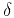Traveltime approximations for transversely isotropic media with an inhomogeneous backgroundNext: The TI eikonal and Up: Alkhalifah: TI traveltimes in Previous: Alkhalifah: TI traveltimes in

# Introduction

The nature of sedimentation and thin layering in the Earth subsurface induces wave propagation characteristics that can be better described by considering the medium to be anisotropic. Specifically, since the layering has a general preferred direction, we find that the transversely isotropic (TI) assumption to be the most practical type of anisotropy to represent big parts of the subsurface. The tilt in this case is naturally set in the direction normal to the layering (Alkhalifah and Sava, 2010; Alkhalifah and Bednar, 2000; Behera and Tsvankin, 2009; Audebert et al., 2006). Thus, this type of model approximates a big portion of the anisotropy resulting from the thin layering. Developing simple traveltime formulations for such a model helps in many applications, including traveltime tomography and integral-based Kirchhoff imaging. The vertical symmetry axis (VTI) medium is a special case of TI in which the symmetry axis is normal to the typically horizontal acquisition surface, and thus results in simpler formulations.

Traveltimes are conventionally evaluated by solving a nonlinear partial differential equation (PDE), better known as the eikonal equation. Among the most known methods for solving this equation are ray tracing and the finite-difference approximations. Finite-difference solutions of the eikonal equation have been recognized as one of the most efficient means of traveltime calculations (van Trier and Symes, 1991; Vidale, 1990; Popovici, 1991; Alkhalifah and Fomel, 2001). Some of main advantages of this method in comparison to ray tracing include the ability to directly provide solutions on regular grids, a complete coverage of the solution space, and a high numerical robustness. On the down side, finite-difference based solutions typically include only the first arrivals, which might not be even the most energetic ones (Cerveny, 2001). In anisotropic media, traveltime computation is dependent on more than one parameter field. However, through careful parametrization of the TI medium,-wave traveltimes in 3D, under the acoustic assumption, become dependent on only three parameter fields and two angles. These parameters include the tilt-direction velocity,, the normal-moveout equivalent velocity,[wherecorresponds to the symmetry direction (Tsvankin, 1997)], and the anellipticity parameter(withalso defined with respect to the symmetry direction). This is evident in the eikonal equation for TI media developed by Alkhalifah (1998,2000a). If the symmetry axis is not vertical, two additional parameters are needed to describe the tilt in 3D, the anglethat the symmetry axis makes with the vertical and the azimuthof the vertical symmetry-axis plane with respect the-axis [Tsvankin (1997)].

The process of finding a stable solution for the TI (or even the VTI) eikonal equation using finite-difference schemes is generally hard, especially since such a process requires finding the root of a quartic equation at each computational step (Wang et al., 2006). However, traveltime computation for a slightly more simplified, but not practical, elliptically anisotropic model is far more efficient. The reason for the high efficiency is that elliptical anisotropy has the same order of complexity (nonlinearity) in the eikonal equation as does the isotropic equation. Thus, though elliptical anisotropy represents an uncommon model in practice, it provides some flexibility in treating the difference between vertical and horizontal velocities, or in other words, the flexibility of stretching the depth axis to obtain accurate reflection depths in imaging (Ohlsen and MacBeth, 1999; Ball, 1995; Peng and Steenson, 2001). However, elliptical anisotropy does not provide accurate focusing for media of typical non-elliptical TI anisotropy (Alkhalifah and Larner, 1994). It will be used here however as the background medium for the perturbation expansions.

The forward problem, whether it is traveltime calculation or wavefield modeling, is a major component of the inversion process. If we model wavefields, we can use those wavefields to generate synthetic data and compare them with measured data as part of what we refer to as wavefield inversion. Likewise, forward traveltime calculation is used to measure the traveltime misfit with those extracted from the data in what is referred to as traveltime tomography. If we assume that one or two of the parameters are constant, the gradient of the objective function with respect to these parameters can be calculated analytically and that usually helps the inversion process. In this paper, I develop simplified formulations for traveltime calculation that can help in resolving anisotropy parameters, specifically the tilt angle.

Alkhalifah (2010) developed an eikonal-based scanning scheme to search for the anisotropy parameterthat can provide the best traveltime fit to the data in a general inhomogeneous background medium. In this earlier paper, I derived first-order linear partial differential equations (PDEs) governing the coefficients of expanding the traveltime solution for VTI media in terms of the independent parameterfrom a background elliptical anisotropic model. I use the Shanks transform [Bender and Orszag (1978)] to enhance the accuracy of the expansion to a point in which the homogeneous-medium versions of it provided exceptional accuracy in describing the traveltime compared to other well-known published moveout equations (Alkhalifah, 2000b). I also suggested a simple angle transformation to make the method work for a tilted symmetry axis with a known tilt direction (i.e. in the direction normal to the layering). However, if the tilt direction is unknown, theestimation will certainly suffer from this limitation.

In this paper, I derive multi-parameter expansions of traveltime as a function of the symmetry-axis angles andwith coefficients estimated using linearized forms of the eikonal equation. The accuracy of such an expansion is again further enhanced using Shanks transform and Pade approximations to obtain higher-order representation. I use a homogeneous-background medium version of the approximation to test its accuracy and then examine the 3D case where the axis direction is described by azimuth as well.Traveltime approximations for transversely isotropic media with an inhomogeneous backgroundNext: The TI eikonal and Up: Alkhalifah: TI traveltimes in Previous: Alkhalifah: TI traveltimes in

2013-04-02